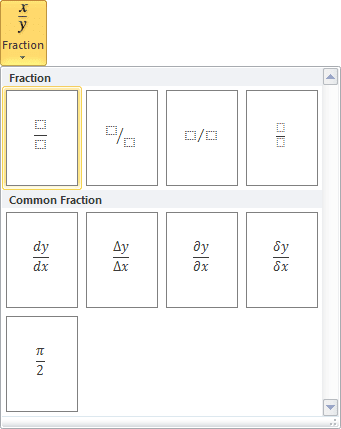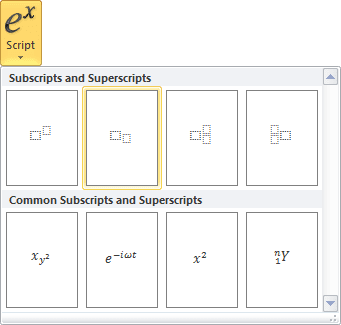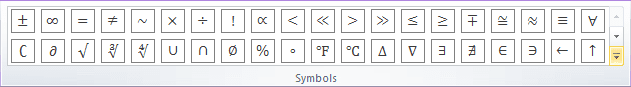# How to insert an equation with integral

Word
This tip display how to insert an equation for example, the Gauss's law, also known as Gauss's flux theorem.

How to add an equation in your document, see Working with Microsoft Equation.

To add integral form of the Gauss's law, do the following:

In the Professional present:

2.   On the Equation Tools Design tab, in the Structures group, click the Integral button:In the Integral list choose Contour Integral:3.   In the integral template:

• In the lower box enter S.
• In the upper box right-click and choose Hide Upper limit in the popup menu:• In the base right box enter E.

4.   On the Equation Tools Design tab, in the Symbols group, in the list of symbols choose.

5.   Then enter dA=.

6.   On the Equation Tools Design tab, in the Structures group, click the Fraction button. In the Fraction list choose Stacked Fraction:7.   Enter Q in the top of your fraction.

8.   In the bottom of your fraction, do the following:

• on the Equation Tools Design tab, in the Structures group, click the Script button.
• in the Script list choose Subscript:• on the Equation Tools Design tab, in the Symbols group, in the list of symbols choose,
• in the lower box of the subscript enter 0.In the Linear present:

2.   Do one of the following:

• On the Equation Tools Design tab, in the Symbols group, click the More button:In the top of list of symbols choose Operators:In the Operators list choose.
• Simply enter \oint.

3.   Enter _S. Then you enter a space key, this linear formula transformed to the professional format.

4.   In the base box of integral, enter E.

5.   On the Equation Tools Design tab, in the Symbols group, choose(or simply enter \bullet).

6.   Then enter dA=Q/, choose(or simply enter \varepsilon) and then _0:Then you enter a space key, your linear formula transformed to the professional format.